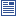Accessibility options:

# Exponential & logarithm functions resources

Show me all resources applicable to

### Community Project (1)Exponential and Logarithm for Economics and Business Studies (SOURCE)
Latex source, image files and metadata for the Fact & Formulae leaflet "Exponential and Logarithm for Economics and Business Studies" contributed to the mathcentre Community Project by Morgiane Richard (University of Aberdeen) and reviewed by Shazia Ahmed (University of Glasgow) and Anthony Cronin (University College Dublin).

### Facts & Formulae Leaflets (1)Exponential and Logarithm for Economics and Business Studies
Overview of the properties of the functions e and ln and their applications in Economics. This leaflet has been contributed to the mathcentre Community Project by Morgiane Richard (University of Aberdeen) and reviewed by Shazia Ahmed (University of Glasgow) and Anthony Cronin (University College Dublin).

### Motivating Mathematics (1)The number 'e' - Trevor Hawkes
Trevor Hawkes discusses the number 'e', its relationship to other numbers - 0, pi and i - and its relevance to everyday life. This resource is released under a Creative Commons license Attribution-Non-Commercial-No Derivative Works and the copyright is held by Skillbank Solutions Ltd.

### Quick Reference (3)The exponential constant e
This leaflet gives some basic information about the constant e. It shows graphs of the exponential function. (Engineering Maths First Aid Kit 3.4)The exponential constant e
The letter e is used in many mathematical calculations to stand for a particular number known as the exponential constant. This leaflet provides information about this important constant, and the related exponential function.The logarithm function
This leaflet provides a table of values and a graph of the logarithm function. (Engineering Maths First Aid Kit 3.7)

### Teach Yourself (1)Exponential and logarithm functions
Exponential functions and logarithm functions are important in both theory and practice. In this unit we look at the graphs of exponential and logarithm functions, and see how they are related.

### Test Yourself (1)Maths EG
Computer-aided assessment of maths, stats and numeracy from GCSE to undergraduate level 2. These resources have been made available under a Creative Common licence by Martin Greenhow and Abdulrahman Kamavi, Brunel University.

### Video (1)Exponential and logarithm functions
Exponential functions and logarithm functions are important in both theory and practice. In this unit we look at the graphs of exponential and logarithm functions, and see how they are related. (Mathtutor Video Tutorial) This resource is released under a Creative Commons license Attribution-Non-Commercial-No Derivative Works and the copyright is held by Skillbank Solutions Ltd.

### Video with captions which require edits (2)Exponential and logarithm functions
Exponential functions and logarithm functions are important in both theory and practice. In this unit we look at the graphs of exponential and logarithm functions, and see how they are related. (Mathtutor Video Tutorial) The video is released under a Creative Commons license Attribution-Non-Commercial-No Derivative Works and the copyright is held by Skillbank Solutions Ltd.The number 'e' - Trevor Hawkes
Trevor Hawkes discusses the number 'e', its relationship to other numbers - 0, pi and i - and its relevance to everyday life. The video is released under a Creative Commons license Attribution-Non-Commercial-No Derivative Works and the copyright is held by Skillbank Solutions Ltd.